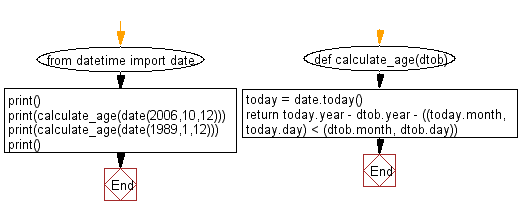﻿ Python: Calculate an age in year - w3resource# Python: Calculate an age in year

## Python Datetime: Exercise-39 with Solution

Write a Python program to calculate an age in year.

Sample Solution:-

Python Code:

``````from datetime import date

def calculate_age(dtob):
today = date.today()
print()
print(calculate_age(date(2006,10,12)))
print(calculate_age(date(1989,1,12)))
print()
```
```

Sample Output:

```10
28
```

Flowchart:## Visualize Python code execution:

The following tool visualize what the computer is doing step-by-step as it executes the said program:

Python Code Editor:

What is the difficulty level of this exercise?

Test your Python skills with w3resource's quiz

﻿

## Python: Tips of the Day

Free up Memory:

• Manual garbage collection can be performed on timely or event based mechanism.
```import gc
collected_objects = gc.collect()
```